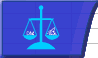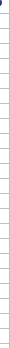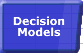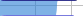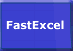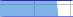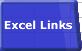# Circular References & Goal SeekSmart Recalculation Evaluation Circumstances Calculation Process Dependency Trees Controlling Calculation "CALCULATE" in Status Bar Calculation Methods Calculating from VBA Volatile Functions & Actions User-Defined Functions Repetitive Calculation Version Timing Comparison## Repetitive Calculation Features and Add-Ins.

Some Excel features and Add-Ins cause Excel to repeatedly recalculate:

• Excel Tables.
• Scenarios.
• Circular References with Iteration enabled.
• Solver.
• Goal Seek.

Make sure when using these features that you really need them, and that you have optimised fully the blocks of formulae that will be repeatedly recalculated.

### Goal Seek

Goal seek triggers a recalculation of all open workbooks on each iteration: so try to have only asingle small fast workbook open when using Goalseek.

### Circular References

Excel's ability to iteratively solve circular reference problems can be useful, but it is easy to over-use.

The default calculation mode for Excel is to disable iteration, so that if you create a circular reference and calculate, Excel detects it and warns you that you have a circular reference. To iteratively solve the circular reference you must turn on iteration (Tools-->Options-->Calculation). Once you have turned iteration ON you will not get any more messages about circular references, and Excel will repeatedly recalculate until it reaches the limit on the number of iterations, or the largest change in value during an iteration is less than the specified max change value.

Note that Excel uses a different calculation process to solve workbooks with circular references. When designing circular reference solutions you may need to take account of the calculation sequence that Excel uses for circular references.

There is one major problem with using circular references: once you have one circular reference it is very difficult to distinguish between mistakes that have created inadvertent circular references and the intentional circular reference. In most circumstances (particularly with financial calculations) it is possible (and desirable) to 'unroll' the circular reference using an extra step. For example, if you want to calculate a cash balance which includes interest on the balance you can use a circular reference where the interest depends on the balance and the balance depends on the interest. This calculates compound interest. You can 'unroll' the calculation by calculating the balance before interest, then calculating the interest (simple or compound) and then the final balance.

### Intentional Circular References

If for some reason you cannot 'unroll' the calculation then I would advise using Stephen Bullen's technique of including an IF statement in your intentional circular references that acts as a switch to turn off the circular references, and sets up the initial condition for the iterative solution.

A1:=1
B1:=IF(A1<>0,C1,A1)
C1:=0.1*E1
D1:=100
E1:=D1+B1

If A1 is zero and iteration is disabled then Excel will not regard this calculation as a circular reference, so any circular reference detected is probably intentional. Set A1 to 1 and enable iteration to request Excel to solve using iteration.

Note that not all circular calculations converge to a stable solution. Another useful piece of advice from Stephen Bullen is to test the calculation in manual calculation with the number of iterations set to 1. Pressing F9 will single-step the calculation so you can watch the behaviour and see if you have genuinely converged to the correct solution.

There are a number of examples of using intentional circular references on Stephen Bullen's website.

### Circular References and Calculation Speed.

Because Excel calculates circular references sheet-by-sheet without considering dependencies you will tend to get very slow calculation if your circular references span more than one worksheet. Try to move the circular calculations onto a single worksheet or optimise the worksheet calculation sequence to avoid unnecessary calculations.

Before the iterative calculations start Excel has to recalculate the workbook to identify all the circular references and their dependents. This process is equivalent to 2 or 3 iterations of the calculation.

After the circular references and their dependents have been identified each iteration requires Excel to calculate not only all the cells in the circular reference, but also any cells which are dependent on the cells in the circular reference chain. So if you have a heavy calculation which is dependent on cells in the circular reference it can be faster to isolate this into a separate closed workbook and open it for recalculation after the circular calculation has converged.

And of course it is important to minimise both the number of cells in the circular calculation and the calculation time taken by these cells.

### Circular References and UDFs.

If you write a UDF that is either in or dependent on the circular chain it will be executed repeatedly. You can detect whether the UDF is being calculated during the first or second step of the calculation process by checking Application.Iteration. This will be False during the first step and True during the second step.© 2001-2020 Decision Models Legal Disclaimer Privacy Statement